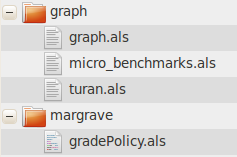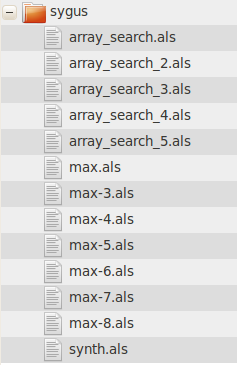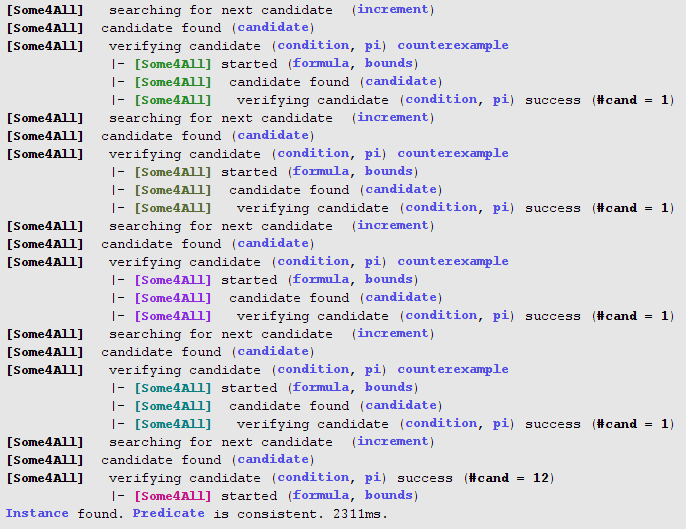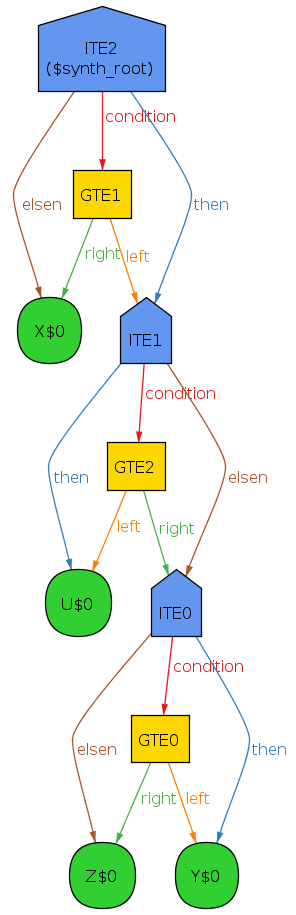# Alloy*: A Higher-Order Relational Constraint Solver

• retains the syntax of Alloy: predicate logic + relational algebra
• permits higher-order quantification: changes the semantics of Alloy only by expanding the set of specifications that can be analyzed
• implements CEGIS on top of Kodkod: all higher-order quantifiers are analyzable (regardless of their position in the formula)
• applications: synthesis, game theory, minimization/maximization, ...
• papers: ICSE 2015, FMSD 2017

hola-0.3 (sources included)
GPLv3
requirements
Java 1.6
```  https://github.com/aleksandarmilicevic/hola/releases/download/v0.3_2018-04-17/hola-0.3_2018-04-17.jar
java -jar hola-0.3_2018-04-17.jar
```

## sample models

sygus
syntax guided program synthesis benchmarks
margrave
Margrave policy synthesizer
graph
various graph algorithms
```  select menu: File -> Open Sample Models
choose path: "models/hol"
```## examples

### Turan's graph theorem

The formulation of Turan's theorem states that a `k+1`-free graph with `n` nodes can maximally have `(k-1)n2/(2k)` edges. A graph is `k+1`-free if it contains no clique with `k+1` nodes (a clique is a subset of nodes in which every two nodes are connected by an edge); this is equivalent to saying that its maximum clique has exactly `k` nodes. A formalization of Turan's theorem in Alloy is given in the figure on the right.

The formalization of the `maxClique` property is higher-order, because it quantifies over all possible sets of `Node`s to assert that there does not exist another set of nodes which is a `clique` and has more nodes than the max-clique. Higher-order specifications are expressible in Alloy, but the official Alloy Analyzer is not powerful enough to analyze them. In Alloy*, in contrast, this check can be automatically performed to confirm that no counterexample can be found within the specified scope.

There are two ways to now formalize Turan's theorem (the `Turan_dom_constr` and `Turan_classical` commands). In both cases, the idea is to asserts that for all possible `edge` relations (from `Node` to `Node`) that are symmetric and irreflexive, if the max-clique in that graph has `k` nodes (`k=#mClq`), the number of selected edges (`e=(#edges).div`) must be at most `(k-1)n2/(2k)` (the number of tuples in `edges` is divided by `2` because the graph in setup of the theorem in undirected). The differences, although syntactic, have significant impact on performance:

classical
the classical way to constrain the domain of a universal quantifier (e.g., all edges that are symmetric and irreflexive) is to use an implication in the quantifier body; similarly, to constraint the domain of an existential quantifier (e.g., some set of nodes that is max-clique), a conjunction in the body is used.
with domain
constraints
for an efficient CEGIS implementation, we prefer domain constraints to be given explicitly (as opposed to merged and inlined in the quantifier body) using the newly introduced `when` clause (not available in standard Alloy).
 command\scope 5 6 7 8 9 10 `Turan_classical` 4s 10s 43s to to to `Turan_dom_constr` 0.3s 3s 6s 10s 44s 170s comparison of analysis times for various scopes
```some sig Node  {}

// between every two nodes there is an edge
pred clique[edges: Node -> Node, clq: set Node] {
all disj n1, n2: clq | n1 -> n2 in edges
}

// no other clique with more nodes
pred maxClique[edges: Node -> Node, clq: set Node] {
clique[edges, clq]
no cq2: set Node | cq2!=clq && clique[edges,cq2] && #cq2>#clq
}

// symmetric and irreflexive
pred edgeProps[edges: Node -> Node] {
(~edges in edges) and (no edges & iden)
}

// max number of edges in a (k+1)-free graph with n
// nodes is (k-1)n^2/(2k)
check Turan_dom_constr {
all edges: Node -> Node when edgeProps[edges] |
some mClq: set Node when maxClique[edges, mClq] |
let n = #Node, k = #mClq, e = (#edges).div |
e <= k.minus.mul[n].mul[n].div.div[k]
}for 7 but 0..294 Int -- checks in ~6s

// same as above, but with inlined domain constraints
check Turan_classical {
all edges: Node -> Node | edgeProps[edges] implies {
some mClq: set Node {
maxClique[edges, mClq]
let n = #Node, k = #mClq, e = (#edges).div |
e <= k.minus.mul[n].mul[n].div.div[k]
}
}
} for 7 but 0..294 Int -- checks in ~43s
```

### program synthesis

#### program ast

```abstract sig Bool {}
one sig BoolTrue extends Bool {}
one sig BoolFalse extends Bool {}

abstract sig Node {}
abstract sig BoolNode extends Node {}
abstract sig IntNode  extends Node {}
abstract sig Var      extends IntNode {}

abstract sig IntLit   extends IntNode { val: one Int }
abstract sig IntComp  extends BoolNode {
left, right: IntNode
}
abstract sig BoolComp extends BoolNode {
left, right: BoolNode
}

sig EQ, GTE, LTE, GT, LT extends IntComp {}
sig And, Or extends BoolComp {}
sig Not     extends BoolNode { arg: BoolNode }
sig ITE     extends IntNode {
condition: BoolNode, then, elsen: IntNode
}```

#### invariants

```pred acyclic[r: univ->univ, s: set univ] {
all x: s | x !in x.^r
}
fact {
acyclic[arg + condition + then + elsen +
IntComp<:left   + IntComp<:right +
BoolComp<:left  + BoolComp<:right, Node]
}```

#### semantics

```pred semantics[eval: Node -> (Int + Bool)] {
all n: ITE {
eval[n] in Int
eval[n.condition] in Bool && eval[n.then] in Int && eval[n.elsen] in Int
eval[n.condition] = BoolTrue implies
eval[n.then] = eval[n] else eval[n.elsen] = eval[n]
}

all n: BoolNode | eval[n] in Bool
all n: IntComp  | eval[n.left] in Int and eval[n.right] in Int
all n: BoolComp | eval[n.left] in Bool and eval[n.right] in Bool
all v: Var      | one eval[v] and eval[v] in Int
all i: IntLit   | one eval[i] and eval[i] in Int and eval[i] = i.val

all n: Not  | eval[n.arg] in Bool
all n: EQ   | eval[n.left] = eval[n.right] implies
eval[n] = BoolTrue else eval[n] = BoolFalse
all n: GTE  | eval[n.left] >= eval[n.right] implies
eval[n] = BoolTrue else eval[n] = BoolFalse
all n: LTE  | eval[n.left] <= eval[n.right] implies
eval[n] = BoolTrue else eval[n] = BoolFalse
all n: GT   | eval[n.left] > eval[n.right] implies
eval[n] = BoolTrue else eval[n] = BoolFalse

all n: LT   | eval[n.left] < eval[n.right] implies
eval[n] = BoolTrue else eval[n] = BoolFalse

all n: And  | (eval[n.left] = BoolTrue and eval[n.right] = BoolTrue) implies
eval[n] = BoolTrue else eval[n] = BoolFalse
all n: Or   | (eval[n.left] = BoolTrue or eval[n.right] = BoolTrue) implies
eval[n] = BoolTrue else eval[n] = BoolFalse
all n: Not  | eval[n.arg] = BoolFalse implies
eval[n] = BoolTrue else eval[n] = BoolFalse
}```

#### specification for max-4

```one sig X, Y, Z, U extends Var {}

pred spec[root: Node, eval: Node -> (Int + Bool)] {
all v: Var | eval[root] >= eval[v]
some v: Var | eval[root] = eval[v]
}```

#### generic synthesis predicate

```pred synth[root: IntNode] {
all env: Var -> one Int |
some eval: Node -> one (Int+Bool) when env in eval && semantics[eval] |
spec[root, eval]
}```

#### solving the synth predicate

`run synth for 10 but 0..3 Int, exactly 3 ITE, exactly 3 GTE`#### generated program## higher-order analysis options

 name type default description Solver MiniSat/SAT4J/... SAT4J SAT solver to use. Selected solver must support incremental solving if higher-order solving is used, i.e., must be one of: MiniSat, SAT4J, Glucose, CriptoMiniSat. Use higher-order solver yes/no no Enable/disable higher-order solver. Create relations for atoms yes/no yes Whether to create a Kodkod relation for each atom; must be set to "yes" when higher-order solving is used. Force incremental CEGIS induction yes/no yes Whether to force incremental solving even when the induction increment formula is not first-order (by using a first-order approximation instead); "yes" typically results in better performance. Maximum number of CEGIS iterations int 100 Maximum number of iterations per each CEGIS loop. Save CEGIS candidates as Viz/Text/Nothing Viz Whether to save intermediate candidate solutions and how: Vizas Alloy XML instance (can be visualized later on) Textas plain text (human readable, but cannot be visualized) Nothingnot saved. Save CEGIS counterexamples as Viz/Text/Nothing Viz Whether to save intermediate counterexamples and how (same as above). Save CEGIS formulas yes/no yes Whether to save intermediate formulas (saved as plain text). Save CEGIS partial instances yes/no yes Whether to save intermediate partial instances (saved as plain text).